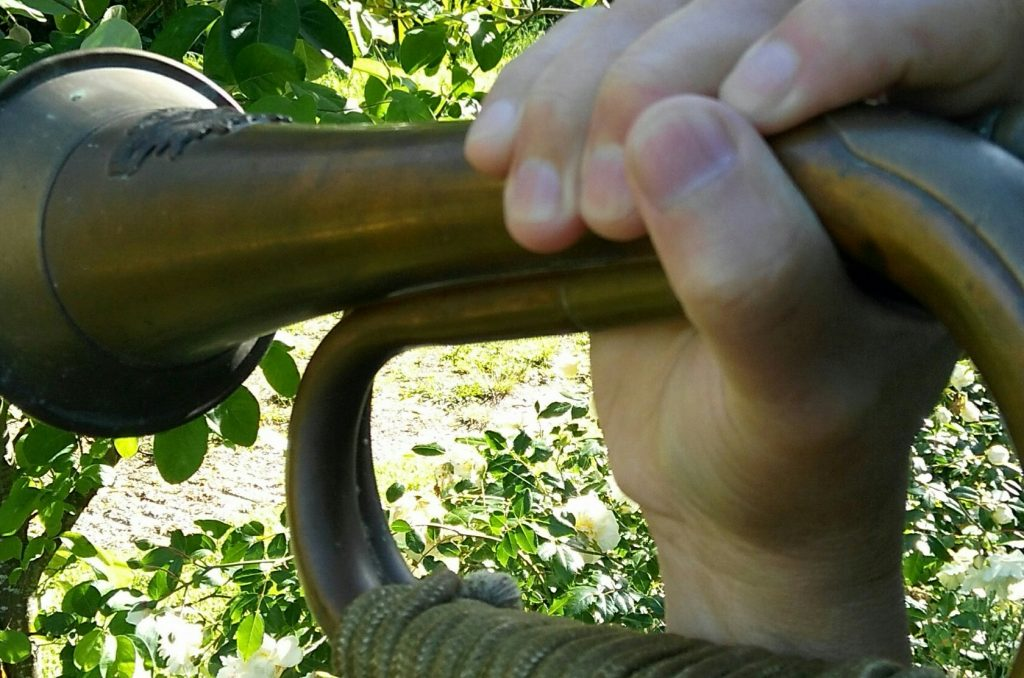## The bugle is one of the simplest brass (or copper) instruments, sounded by the vibration of the lips against a mouthpiece.

All bugle calls consist of basically five notes CG^C^E^G (C = middle C, ^C = higher C as in piano below). These notes are known as the bugle scale. We also use 1,2,3,4,5 to represent the notes to assist beginners in all bugle calls we post on this website as the traditional music notes is not easy to read. Listening and practice is the best way to master bugle as most bugle calls are short and memorable.

The bugle is used mainly in the military, boys’ brigade and boy scouts. Historically the bugle was used to relay instructions from officers to soldiers during battle and to give marching orders to the camps.

You will learn how to play a number of bugle calls. Notes are changed by blowing faster air for higher notes, or slower air for lower notes. With practice the bugle can be mastered quite easily and it is easier than trumpet. Here we go. Let’s try our first bugle call now.One way to hold a bugle

## A plastic bugle

A cheap version of bugle for beginners. It sounds great, light and easy for younger beginners.

## Bugle call – last post

12, 12, 1222342222, 45435435432222,

5432432432433243, 2233443,

245432222, 223333432233343, 2234343,

245432222, 5435432222,

12, 12, 12223, 4.

## Bugle call – second dinner call

^E^G^E^C^G^E^CG^C^C^EG^C^C,

^E^G^E^CG^E^CG^C^C^E^C.

45435432334233,

454354323343.

## Bugle call – fatigue

G^C^EG^C, ^EG^CG^EG^CG^EG^C,

^EG^C^EG^CG^EG^C.

23423, 42324232423,

4234232423.

## Bugle call – reveille rouse

G^CG^C, G^CG^C, G^CG^C^E, REPEAT,

^C^C^E, ^G^E^CG^C^E.

2323, 2323, 23234, REPEAT,

334, 543234.

## Bugle call – rations

^C^C^C^C^C^E^C^E^G^E^C^E^G^E^C^C^C^C^C.

333334345434543333.

## Bugle call – quarter bugle

^E^E^C^E^CG^C^E^C.

443432343.

## Bugle call – parade for guard

^G^E^CG^C^EG, ^G^E^CG^C. Repeat.

5432342, 54323. Repeat.

## Bugle call – first dinner call

G^C^C^C^C^EG, G^C^C^C^C^EG. Repeat.

2333342, 2333342. Repeat.

## Bugle call – fire alarm

^G^G^G^E^E^E^C^C^C, ^C^CGG^C^CGG^C^CGG^C.

555444333, 3322332233223.

## Bugle call – dismiss or no parade

G^C^C^C^E^C, G^C^C^C^E^C,

G^C^C^C^E^C^C^CG^C^E^G^E^C.

233343, 233343, 23334333234543.